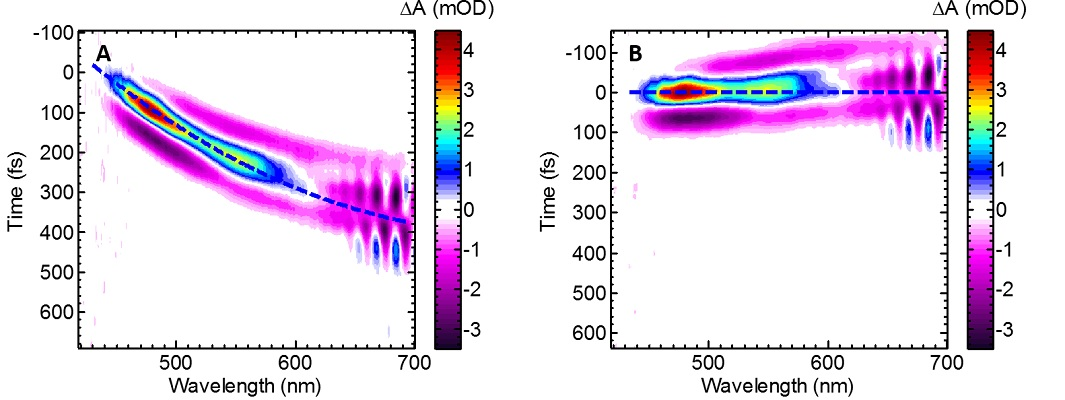Sale!

# MATLAB code for remove chirp from a data set

17

## Description

The maximum of each wavelength slice of the coherent spike resulting from cross correlation is found. Then a polynomial is t to these maxima. Each wavelength slice is shifted such that it’s time zero matches the first slice’s time zero.

In practice, the calculated shift for each wavelength slice may be smaller than the step size that the data was collected at. Thus, before shifting, the data set is resampled at a higher frequencyOnce the time shifts have been applied to the data resampled again to the original experimental sampling frequency.https://matlab1.com/shop/matlab-code/matlab-code-to-extract-the-total-cycling-time-and-frequency-of-clamping/

https://arxiv.org/ftp/arxiv/papers/1502/1502.03628.pdf

## Reviews

There are no reviews yet.

Category: